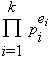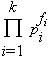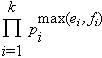# least common multiple

The least common multiple of two (or more) nonzero integers is the least positive integer divisible by all of them. This is usually denoted lcm. For example, lcm(-12,30)=60. Notice

Note that we can use the first of these, and the Euclidean algorithm, to find the least common multiple without factoring. However, if we know the prime factorization of a is, and that of b is, then lcm(a,b) is.

We can generalize gcd(a,b).lcm(a,b) = ab to three variables in a number of ways:

• gcd(a,b,c).lcm(ab,ac,bc) = abc
• lcm(a,b,c).gcd(ab,ac,bc) = abc
• gcd(lcm(a,b),lcm(a,c),lcm(b,c)) = lcm(gcd(a,b), gcd(a,c),gcd(b,c))

Finally, as a direct result of the properties of min and max functions we have the dual relations:

• gcd(a, lcm(b,c)) = lcm(gcd(a,b),gcd(a,c))
• lcm(a, gcd(b,c)) = gcd(lcm(a,b),lcm(a,c))